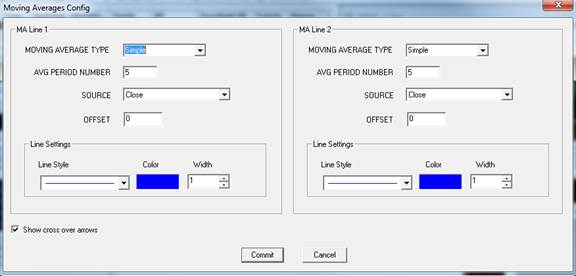# Moving Average Pair

User can add Moving Average Pair indicator to their chart by right click the chart and select Study Config to open the study configure window first, then select the “Moving Average Pair” from the study list and add it into the Studies In Chart field.Moving Averages Configure window allows user to modify the Moving Average Pair chart, such as change the moving average types and line colors. User can open this Moving Averages Configure window either by click the “Config” button or double click the study “Moving Average Pair” under Studies In Chart section.

## Explanation of Each Field

·         MA Line 1 and MA Line 2: Allows user to drawn two moving average lines simultaneously and do the comparison between them.

·         Moving Average Type: User can use the dropdown menu to change the moving average type:

Ø  Simple: Selected this to use the simple moving average.

Ø  Weighted: Selected this to use the weighted moving average.

Ø  Exponential: Selected this to use the exponential moving average.

Ø  Smooth: Selected this to use the smooth moving average.

Ø  Volume Weighted: Selected this to use the volume weighted moving average.

·         AVG Period Number: Enter the study period for the selected moving average.

·         Source: Use the dropdown menu to select the study source for the selected moving average type.

Ø  Open: Use the open price data to calculate the moving average.

Ø  High: Use the highest price data to calculate the moving average.

Ø  Low: Use the lowest price data to calculate the moving average.

Ø  Close: Use the close price data to calculate the moving average.

Ø  (High+Low)/2: Use this equation to calculate the price first, then use those price data to calculate the moving average.

Ø  (High+Low+Close)/3: Use this equation to calculate the price first, then use those price data to calculate the moving average.

Ø  (Open+High+Low+Close)/4: Use this equation to calculate the price first, then use those price data to calculate the moving average.

·         Offset: Allows user to view the offset chart.

·         Calculate On Volume: Allows user to calculate the moving average base on the volume instead of price.

·         Line Settings: Allows user to modify the moving average line.

Ø  Line Style: Allows user to use the dropdown menu to change the line style.

Ø  Color: Click on the color field allows user to change the line color.

Ø  Width: Allows user to adjust the line’s width.

·         Show cross over arrows: Check off this box to add an arrow when there is a crossover/intersection between two moving average lines.